Unit 2 quiz period 3
Email *
Last Name *
First Name *
Period *
1. What is the maximum number of covalent bonds than an atom of Nitrogen can form?
2 points
2. Use the electron dot diagrams below to answer the question. What is the true identity of element "Y"
2 points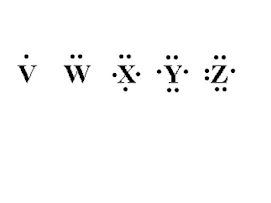3. Use the electron dot diagrams below to answer the question. If X and V were to covalently bond to each other, what molecule would result?
2 points4. Which of the following molecules could be built using ONLY single bonds?
2 points
5. The chemical properties of an element are determined by its:
2 points
6. Sulfur has an atomic number of 16. Which of the following elements would you expect to be most similar to sulfur in terms of its chemical properties?
2 points
7. Below (diagram 1) is the Bohr model for a particular element. What is the correct lewis dot diagram for this element?
2 points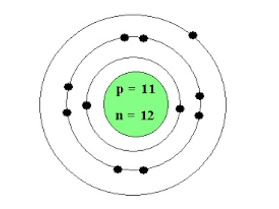8. What would be the correct ionic compound if Barium (Ba) transferred electrons to (Cl) chlorine?
2 points
9. Potassium (K), atomic number 19, will MOST likely react with which of the following element to form an ionic bond?
2 points
10. Which of the following identifies the number and location of protons in a lithium atom?
0 points
11. The figure below is the structural formula an organic molecule. What is it's correct molecular formula?
2 points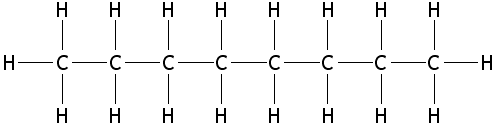12. In question 11 above, what is the molecule illustrated called?
2 points
13. A covalent bond occurs when
2 points
14. Refer to diagram below. Which of the following molecules IS built correctly?
2 points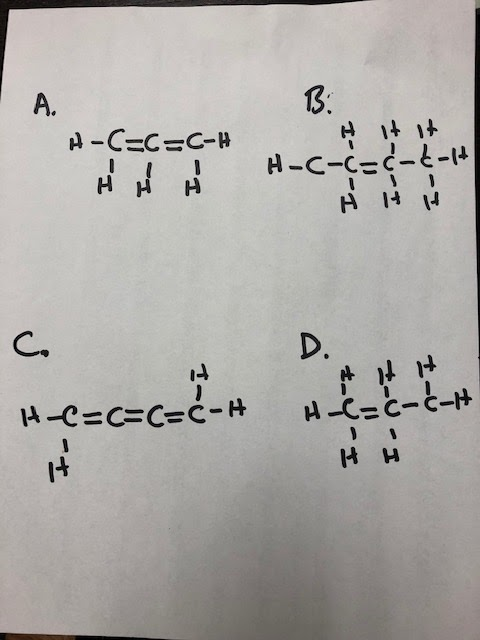15. The molecules below, 1- butyne and 2-butyne are called
2 points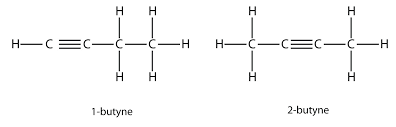15. The molecular formula (s) for the molecules below would be: check ALL that apply
2 points16. Study the table below. Which element would be considered an ion? Check all that apply
2 points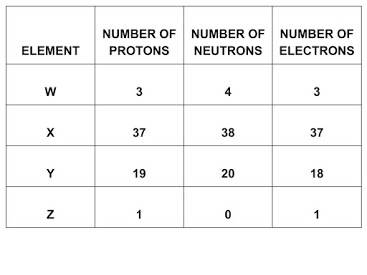17. Electrons are transferred from one element to another in a (an)
2 points
18. The gasoline consumed by an automobile is a fossil fuel consisting mostly of
2 points